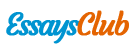# Multiple Regression Analysis on Store Sites

Autor:   •  November 27, 2017  •  1,140 Words (5 Pages)  •  242 Views

Page 1 of 5

...

The overall picture is that stores in sites with higher percentage in inc10-14 and higher median home value and greater population and bigger selling size are likely to have higher sales.

Now we want to see whether the “competitive type” is better at forecasting than demographic analysis. Since competitive type is not quantitative, firstly, we convert competitive type into dummy variables which equals one for the category and zero otherwise. To better examine the relationship between each comtype and average sales, we draw a pivot table. We find that the average sales of comtype 3,4,5 and 6 are very close. We can omit comtype 3,4,5,6 and leave them as baseline.

[pic 10]

Figure 4. Pivot table of sales and comtype

We get the following regression summary Figure 5 from running stepwise procedures.

[pic 11]

Figure 5 Summary of regression using comtype

And also, draw residuals scatter plot and histogram Figure 6 to check the technical assumption.

[pic 14][pic 15][pic 12][pic 13]

Figure 6 Analysis of residuals

The residuals generally follow a normal distribution and there is no obvious pattern in it.

Conclusion

This new regression Forecasted sales=22744+22013*comtype1+10337*comtype2-7952*comtype7+51*selling_sqrft, which has an R2 of 0.66 and SE of 6523 tells us that the variations in selling_sqrft and comtype 1, 2 and 7 can explain about 66% of the variation in sales. And the forecasts using this regression equation generally are around \$6523 from the actual sales figures and almost are within 2*6523=\$13046. The second regression mode has higher R2 and lower SE. So the comtype approach is better than demographic analysis in forecasting the sales.

From the pivot table we know the average sales of comtype 3,4,5 and 6 are very close. So we can rule out 3 of them and just retain 1 to simplify future study.

The potential new store sites A and B under consideration has selling_sqrft of 125k and 120k respectively and comtype 1 and 5 respectively. Since the new regression equation is more accurate in forecasting, we use this model. We get that for A site the forecasted sales is \$51,132,000. For B site the forecasted sales is \$28,864,000. So we would recommend site A.

We could see from the regression equation that selling_sqrft is positive related with sales, increase in selling_sqft is associated with increase in sales. But percentage hard goods stocked is insignificant in affecting sales according to the regression model. That is because consumers seldom care about the margins on goods.

1.2.3. Pekoz, Custom 2014 Edition, 232-234, 407-409.

...